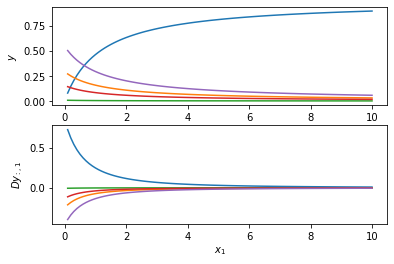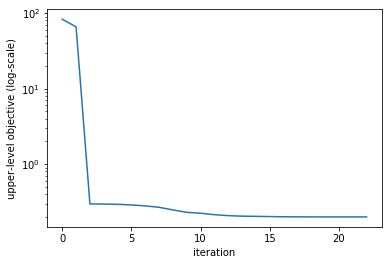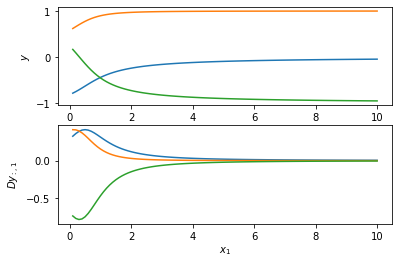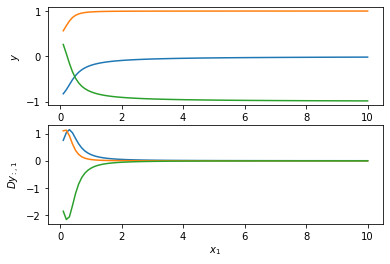# Implementing a Declarative Node using the ddn.basic.node Module¶

In this notebook we demonstrate how to implement a declarative node using the ddn.basic.node module. This will allow us to explore the behavior of the node and solve simple bi-level optimization problems. For more sophisticated problems and integrating into large deep learning models use modules in the package ddn.pytorch instead.

We consider the problem of minimizing the KL-divergence between the input $x$ and output $y$ subject to the output forming a valid probablility vector (i.e., the elements of $y$ be positive and sum to one). We will assume strictly positive $x$. The problem can be written formally as

$$\begin{array}{rll} y =& \text{argmin}_u & - \sum_{i=1}^{n} x_i \log u_i \\ & \text{subject to} & \sum_{i=1}^{n} u_i = 1 \end{array}$$

where the positivity constraint on $y$ is automatically satisfied by the domain of the log function.

A nice feature of this problem is that we can solve it in closed-form as $$y = \frac{1}{\sum_{i=1}^{n} x_i} x.$$

However, we will only use this for verification and pretend for now that we do not have a closed-form solution. Instead we will make use of the scipy.optimize module to solve the problem via an iterative method. Deriving our deep declarative node from the LinEqConstDeclarativeNode class, we will need to implement two functions: the objective function and the solve function (the constraint and gradient functions are implemented for us).

In :
import numpy as np
import scipy.optimize as opt

import sys
sys.path.append("../")
from ddn.basic.node import *

import warnings
warnings.filterwarnings('ignore')

# create the example node
class MinKLNode(LinEqConstDeclarativeNode):
def __init__(self, n):
# Here we establish the linear equality constraint, Au = b. Since we want the sum of the
# u_i to equal one we set A to be the all-ones row vector and b to be the scalar 1.
super().__init__(n, n, np.ones((1,n)), np.ones((1,1)))

def objective(self, x, u):
return -1.0 * np.dot(x, np.log(u))

def solve(self, x):
# Solve the constrained optimization problem using scipy's built-in minimize function. Here we
# initialize the solver at the uniform distribution.
u0 = np.ones((self.dim_y,)) / self.dim_y
result = opt.minimize(lambda u: self.objective(x, u), u0,
constraints={'type': 'eq', 'fun': lambda u: (np.dot(self.A, u) - self.b)})

# The solve function must always return two arguments, the solution and context (i.e., cached values needed
# for computing the gradient). In the case of linearly constrained problems we do not need the dual solution
# in computing the gradient so we return None for context.
return result.x, None

In :
# test the node
node = MinKLNode(5)
x = np.random.random(5)
print("Input:           {}".format(x))
print("Expected output: {}".format(x / np.sum(x)))

y, _ = node.solve(x)
print("Actual output:   {}".format(y))

Input:           [0.37433256 0.34202705 0.00900571 0.18080674 0.63722868]
Expected output: [0.2425375  0.22160612 0.00583498 0.11714828 0.41287312]
Actual output:   [0.24256142 0.22163053 0.00583478 0.11714742 0.41282586]


We now plot the function and gradient sweeping the first component of the input $x_1$ from 0.1 to 10.0 while holding the other elements of $x$ constant.

In :
%matplotlib inline
import matplotlib.pyplot as plt

x_data = np.linspace(0.1, 10.0, 100)
y_data = []
Dy_data = []
for x in x_data:
y, _ = node.solve(x)
y_data.append(y)

fig = plt.figure()
plt.subplot(2, 1, 1)
plt.plot(x_data, y_data)
plt.ylabel(r"$y$")

plt.subplot(2, 1, 2)
plt.plot(x_data, Dy_data)
plt.xlabel(r"$x_1$"); plt.ylabel(r"$Dy_{:,1}$")
plt.show()### Bi-level optimization¶

Now let's see whether we can use the node within a bi-level optimization problem. We will attempt to learn an input $x$ that results in an output $y$ with smallest norm-squared. Moreover, we will regularize the norm of $x$ to be close to 10. Given our understanding of KL-divergence this should learn a vector $x$ that is a constant multiple of the ones vector (i.e., all elements of $x$ should be the same). Let's see what happens.

In :
from autograd import grad

# define the upper-level objective
def JofXandY(x, y):
"""Computes our upper-level objective given both x and y."""
return np.dot(y, y) + np.power(np.sqrt(np.dot(x, x)) - 10.0, 2.0)

def JofX(x):
"""Computes our upper-level objective given x and with a y that minimizes the lower-level objective."""
y, ctx = node.solve(x)
return JofXandY(x, y)

def dJofX(x):
"""Computes the gradient of the upper-level objective with respect to x."""
y, ctx = node.solve(x)
return Jx(x, y) + np.dot(Jy(x, y), node.gradient(x, y, ctx))

# solve using L-BFGS
x0 = np.random.random(node.dim_x)
history = [JofX(x0)]
result = opt.minimize(JofX, x0, args=(), method='L-BFGS-B', jac=dJofX,
options={'maxiter': 100, 'disp': False},
bounds=[(1.0e-6, None) for xk in x0],
callback=lambda xk : history.append(JofX(xk)))

x = result.x
y, _ = node.solve(x)
print("Found x = {} with norm {:0.2f}".format(x, np.sqrt(np.dot(x, x))))
print("Results in y = {}".format(y))

fig = plt.figure()
plt.semilogy(history)
plt.ylabel("upper-level objective (log-scale)"); plt.xlabel("iteration")
plt.show()

Found x = [4.47086992 4.47239585 4.47174323 4.47191239 4.47375868] with norm 10.00
Results in y = [0.1999367  0.20001299 0.19998036 0.19998882 0.20008113]## Multiple Equality Constraints¶

We can also solve problems with non-linear constraints. If there is just one constraint use EqConstDeclarativeNode as the base class for implementing the node. Otherwise use MultiEqConstDeclarativeNode when there is more than one (non-linear) equality constraint. Consider the following problem with $x, y \in \mathbb{R}^3$.

$$\begin{array}{rll} y =& \text{argmin}_u & \sum_{i=1}^{3} x_i u_i^{2} \\ & \text{subject to} & \sum_{i=1}^{2} u_i^2 = 1 \\ & & \sum_{i=1}^{3} u_i = 0 \end{array}$$

We need to implement three functions: the objective, constraint and solve functions.

In :
# create the example node
# by Suikei Wong (2020)

class MinMulNode(MultiEqConstDeclarativeNode):
def __init__(self):
super().__init__(3, 3)

def objective(self, x, u):
return np.dot(x, u ** 2)

def constraint(self, x, u):
"""Return 2-vector, one element for each constraint."""
return np.array([u ** 2 + u ** 2 - 1, u + u + u])

def solve(self, x):
# Solve the constrained optimization problem using scipy's built-in minimize function.
con1 = {'type': 'eq', 'fun': lambda u: u ** 2 + u ** 2 - 1}
con2 = {'type': 'eq', 'fun': lambda u: u + u + u}
cons = ([con1, con2])
# Initialize the solver at (sin30, cos30, -sin30-cos30) which is a feasible point
u0 = np.array([1/2, np.sqrt(3)/2, -(np.sqrt(3)-1)/2])
result = opt.minimize(lambda u: self.objective(x, u), u0, constraints=cons)

# The solve function must always return two arguments, the solution and context (i.e., cached values needed
# for computing the gradient). In the case of linearly constrained problems we do not need the dual solution
# in computing the gradient so we return None for context.
return result.x, None

In :
# test the node
mul_node = MinMulNode()
x = np.random.random(mul_node.dim_x)
print("Input:           {}".format(x))

y, _ = mul_node.solve(x)
print("Actual output:   {}".format(y))
print("Objective:       {}".format(mul_node.objective(x, y)))
print("Constraints:     {}".format(mul_node.constraint(x, y)))

Input:           [0.50740436 0.31461995 0.4479928 ]
Actual output:   [-0.62835421  0.77792742 -0.14957321]
Objective:       0.4007594124656046
Constraints:     [8.33840210e-08 2.77555756e-17]

In :
# plot the function and gradients
x_data = np.linspace(0.1, 10.0, 100)
y_data = []
Dy_data = []
for x in x_data:
y, _ = mul_node.solve(x)
y_data.append(y)

fig = plt.figure()
plt.subplot(2, 1, 1)
plt.plot(x_data, y_data)
plt.ylabel(r"$y$")

plt.subplot(2, 1, 2)
plt.plot(x_data, Dy_data)
plt.xlabel(r"$x_1$"); plt.ylabel(r"$Dy_{:,1}$")
plt.show()### Equality and Inequality Constraints¶

We now consider a problem with multiple equality and inequality constraints,

$$\begin{array}{rll} y =& \text{argmin}_u & \sum_{i=1}^{3} x_i u_i^{2} \\ & \text{subject to} & \sum_{i=1}^{2} u_i^2 = 1 \\ & & \sum_{i=1}^{3} u_i = 0 \\ & & u_1 - u_2 \leq 0 \end{array}$$

We will construct the problem by deriving from the GeneralConstDeclarativeNode class from ddn.basic.node.

In :
# An example of a simple general declarative node
# by Suikei Wong (2020)

class SimpleGeneralNode(GeneralConstDeclarativeNode):
def __init__(self):
super().__init__(3, 3)

def objective(self, x, u):
return np.dot(x, u ** 2)

def eq_constraints(self, x, u):
return np.array([u ** 2 + u ** 2 - 1, u + u + u])

def ineq_constraints(self, x, u):
return np.array([u - u])

def solve(self, x):
# Solve the constrained optimization problem using scipy's built-in minimize function. Here we
# initialize the solver at the uniform distribution.
con1 = {'type': 'eq', 'fun': lambda u: u ** 2 + u ** 2 - 1}
con2 = {'type': 'eq', 'fun': lambda u: u + u + u}
con3 = {'type': 'ineq', 'fun': lambda u: u - u}

cons = ([con1, con2, con3])
# initialize u0 = [sin30, cos30, -sin30-cos30] which is a feasible point
u0 = np.array([1/2, np.sqrt(3)/2, -(np.sqrt(3)-1)/2])
result = opt.minimize(lambda u: self.objective(x, u), u0, method='SLSQP', constraints=cons)

# The solve function must always return two arguments, the solution and context (i.e., cached values needed
# for computing the gradient). In the case of linearly constrained problems we do not need the dual solution
# in computing the gradient so we return None for context.
return result.x, None

In :
# test the node
gen_node = SimpleGeneralNode()
x = np.random.random(gen_node.dim_x)
print("Input:           {}".format(x))

y, _ = mul_node.solve(x)
print("Actual output:   {}".format(y))
print("Objective:       {}".format(gen_node.objective(x, y)))
print("Eq. Constraints: {}".format(gen_node.eq_constraints(x, y)))
print("Ineq. Consts:    {}".format(gen_node.ineq_constraints(x, y)))

Input:           [0.19007718 0.22730736 0.16264973]
Actual output:   [-0.74622598  0.66569272  0.08053327]
Objective:       0.2076304951190502
Eq. Constraints: [6.45243792e-09 5.55111512e-17]
Ineq. Consts:    [-1.4119187]

In :
x_data = np.linspace(0.1, 10.0, 100)
y_data = []
Dy_data = []
for x in x_data:
y, _ = gen_node.solve(x)
y_data.append(y)

fig = plt.figure()
plt.subplot(2, 1, 1)
plt.plot(x_data, y_data)
plt.ylabel(r"$y$")

plt.subplot(2, 1, 2)
plt.plot(x_data, Dy_data)
plt.xlabel(r"$x_1$"); plt.ylabel(r"$Dy_{:,1}$")
plt.show()In [ ]: